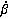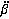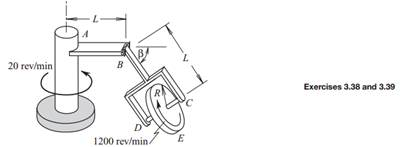### Create an Account

Home / Questions / The disk spins about its axis CD at 1200 revmin as the system rotates about the vertical a...

# The disk spins about its axis CD at 1200 revmin as the system rotates about the vertical axis at 20 revmin Both rates are constant The angle of elevation of the arm supporting the disk is such

The disk spins about its axis CD at 1200 rev/min as the system rotates about the vertical axis at 20 rev/min. Both rates are constant. The angle of elevation of the arm supporting the disk is such that= 10 rad/s and= −500 rad/s2 when β = 36.87°. Determine the velocity and acceleration of point E, which is the lowest point on the perimeter of the disk.Jun 16 2020 View more View LessSubscribe To Get Solution